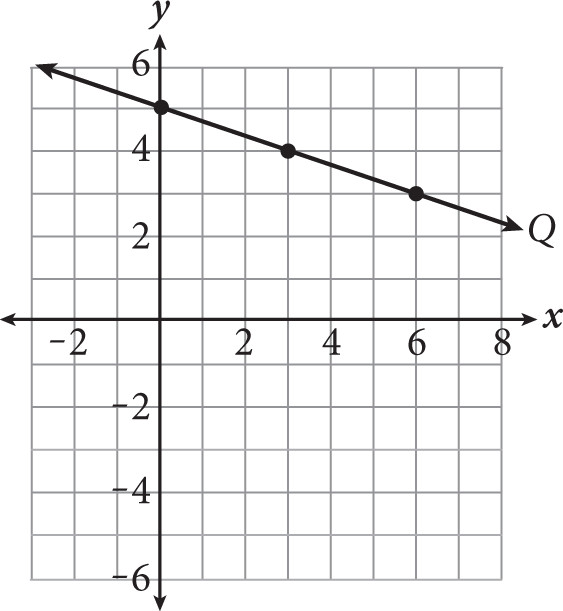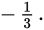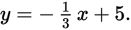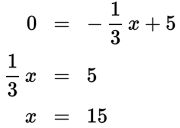# SAT Math Multiple Choice Question 363: Answer and Explanation

### Test Information

Question: 363

3.Where will line Q shown in the graph intersect the x-axis?

• A. 13
• B. 14
• C. 15
• D. 16

Explanation:

C

Difficulty: Easy

Category: Heart of Algebra / Linear Equations

Strategic Advice: Finding an x-intercept is easy when you know the equation of the line—it's the value of x when y is 0.

Getting to the Answer: Everything you need to write the equation is shown on the graph. The y-intercept is 5 and the line falls 1 unit and runs 3 units from one point to the next, so the slope isThis means the equation of the line, in slope-intercept form, isNow, set the equation equal to zero and solve for x:Line Q will intercept the x-axis at 15.